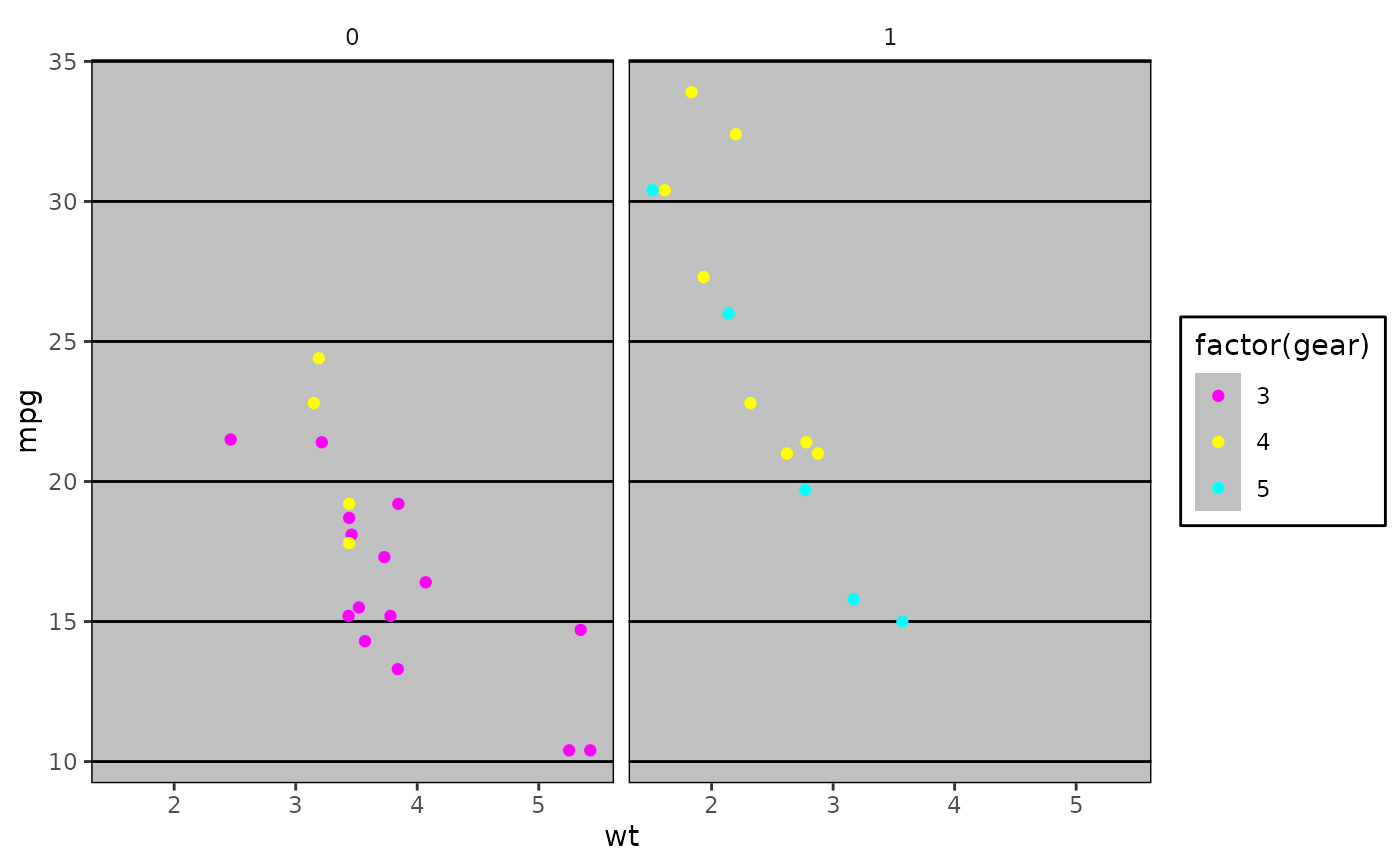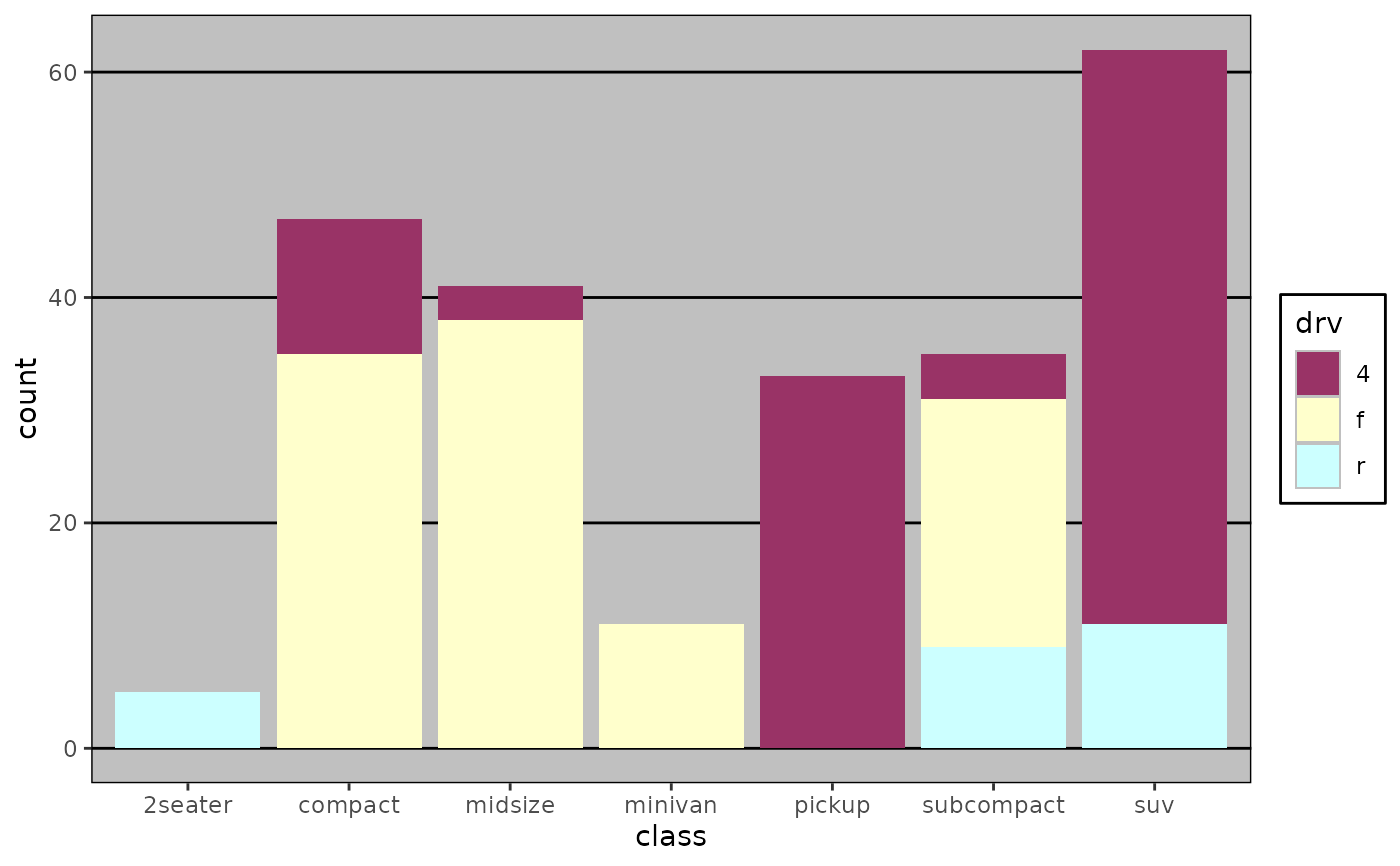Theme to replicate the ugly monstrosity that was the old gray-background Excel chart. Please never use this. This theme should be combined with the scale_colour_excel() color scale.

theme_excel(base_size = 12, base_family = "", horizontal = TRUE)

## Arguments

base_size base font size, given in pts. base font family logical. Horizontal axis lines?

## Value

An object of class theme().

Other themes excel: theme_excel_new()

## Examples

library("ggplot2")

# Line and scatter plot colors
p <- ggplot(mtcars) +
geom_point(aes(x = wt, y = mpg, colour = factor(gear))) +
facet_wrap(~am)
p + theme_excel() + scale_colour_excel()# Bar plot (area/fill) colors
ggplot(mpg, aes(x = class, fill = drv)) +
geom_bar() +
scale_fill_excel() +
theme_excel()